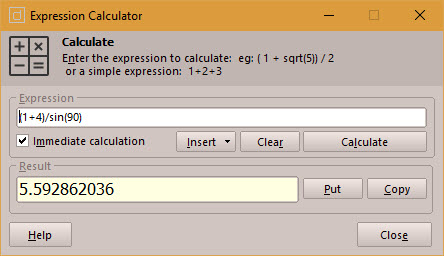# Expression CalculatorWith the doogiePIM Expression Calculator you can perform simple or complex calculations. Rather than using the traditional desktop style calculator, in the BiteSpire Expression Calculator, you would enter the expression in the input box in one line.

Enter an expression, such as:

10+45+112+99456-443

or

cos(90)

To see an immediate answer while typing the expression, place a checkmark next to "Immediate calculation".

The command buttons are:

"Insert", to quickly insert mathematical functions, like cos, sin, log etc.

"Clear" button command will clear the input box.

"Calculate" performs the calculation and displays the result in the yellow area.

"Put" copies the result into the Calculate input box

"Copy" Copies the result to the clipboard.

Functions

pi

Returns pi as 3.141592654

exp

the value of e raised to the power of x

if(x,y,z)

if x=True(or 1) then return y else return z

randg

Draw from normal distrib. (mean=x, sd =y)

nan

Not a number, mathematical error in result

isnan

Is Not a Number (has error)?

random

random number between 0 and 1

sqr

the square of a number (x*x)

sqrt

the square root of a number

abs

Absolute value.

round

Round to the nearest integer.

trunc

Truncates a real number to an integer.

ln

Natural logarithm of x.

log10

Logarithm base 10 of x.

logN

Logarithm base x of y.

power

Raise to the power: x^y.

pow

Power: x^y.

intpower

Integer power: x^y.

max

The maximum of both arguments.

Min

The minimum of both arguments.

sin

Sine of an angle in radians.

cos

Cosine of an angle in radians.

tan

Tangent of an angle in radians.

arcsin

arccos

arctan2

arctan

sinh

Hyperbolic sine of an angle in radians.

cosh

Hyperbolic sine of an angle in radians

tanh

Hyperbolic tangent of an angle in radians

arcsinh

arccosh

arctanh

pos

Position in of substring in string.

Operands

comparison:  >   <   <>   =   <=   >=   (also works on strings)

logical: and or xor not

factorial: !

percentage: %

assign to variables: :=

The required format of the expression is Pascal style with the following additional operands:

- factorial (x!)

- power (x^y)

- percentage (x%)

Implicit multiplying is not supported: e.g.

(X+1)(24-3)

generates a syntax error and should be replaced with:

(x+1)*(24-3)

Logical functions evaluate in 0 if False and 1 if True.

Precedence

The precedence of the operands is little different, giving a lower precedence to logical operands, as these only act on Booleans (and not on integers like in Pascal).

1 (highest): ! -x +x %

2: ^

3: * / div mod

4: + -

5: > >= < <= <> =

6: not

7: or and xor

8: (lowest): :=

Related Topics